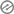# Compute the standard deviation of the following scores 6, 7, 6, 9, 5, 2, 6, 7, 3, 4

The steps are as follows:

1. Calculate the mean (average) of the scores.
2. Subtract the mean from each score, square the result.
3.4. Calculate the mean of those squared differences.
5. Take the square root of that mean to get the standard deviation.Step 1: The mean is indeed 5.5.

Step 2: Subtract the mean from each score, square the result:

(6 – 5.5)^2 = 0.25(7 – 5.5)^2 = 2.25

(6 – 5.5)^2 = 0.25

(9 – 5.5)^2 = 12.25(5 – 5.5)^2 = 0.25

(2 – 5.5)^2 = 12.25

(6 – 5.5)^2 = 0.25(7 – 5.5)^2 = 2.25

(3 – 5.5)^2 = 6.25

(4 – 5.5)^2 = 2.25Step 3: Calculate the mean of those squared differences: (0.25 + 2.25 + 0.25 + 12.25 + 0.25 + 12.25 + 0.25 + 2.25 + 6.25 + 2.25) / 10 = 3.85.

Step 4: Take the square root of the mean: √3.85 ≈ 1.96.So, the corrected standard deviation of the given scores is approximately 1.96.

[mediator_tech]Identify and expatiate on any five (5) main areas of education that were identified as crucial by the 1969 National Curriculum Conference.

Evaluation

1. The given scores are: 6, 7, 6, 9, 5, 2, 6, 7, 3, and 4. Compute the standard deviation.

(a) 2.19
(b) 1.96
(c) 3.85

2. What is the mean of the scores 6, 7, 6, 9, 5, 2, 6, 7, 3, and 4?(a) 5.9
(b) 5.5
(c) 6.4

3. To calculate the standard deviation, one needs to square the differences between each score and the _____.

(a) Mean
(b) Median
(c) Mode4. After squaring the differences, the next step is to calculate the mean of these squared differences, which is known as the _____.

(a) Variance
(b) Median
(c) Range

5. The square root of the mean of squared differences gives us the _____.(a) Mean Absolute Deviation
(b) Standard Deviation
(c) Variance

6. In the given scores, which number appears most frequently?

(a) 7
(b) 6
(c) 47. A higher standard deviation indicates that the data points are _____ from the mean.

(a) Closer
(b) Farther
(c) Unrelated

8. If all the scores in a dataset are the same, the standard deviation would be _____.(a) 0
(b) 1
(c) Equal to the mean

9. Which measure of central tendency is affected by outliers and can consequently impact the standard deviation?

(a) Mean
(b) Median
(c) Mode10. The standard deviation provides information about the _____ of the data.

(b) Central value
(c) Skewness

11. Which step involves summing the squared differences between each score and the mean?(a) Step 1
(b) Step 2
(c) Step 3

12. The standard deviation is always non-negative because it involves _____.

(b) Subtraction
(c) Squaring

13. Out of the given scores, which number has the greatest influence on the standard deviation due to its larger distance from the mean?

(a) 2
(b) 7
(c) 4

14. The standard deviation is a measure of the _____ of data values around the mean.

(a) Variability
(b) Uniqueness
(c) Rarity

15. Which statistical term describes the average squared difference between each data point and the mean?

(a) Variance
(b) Mean Absolute Deviation
(c) Range